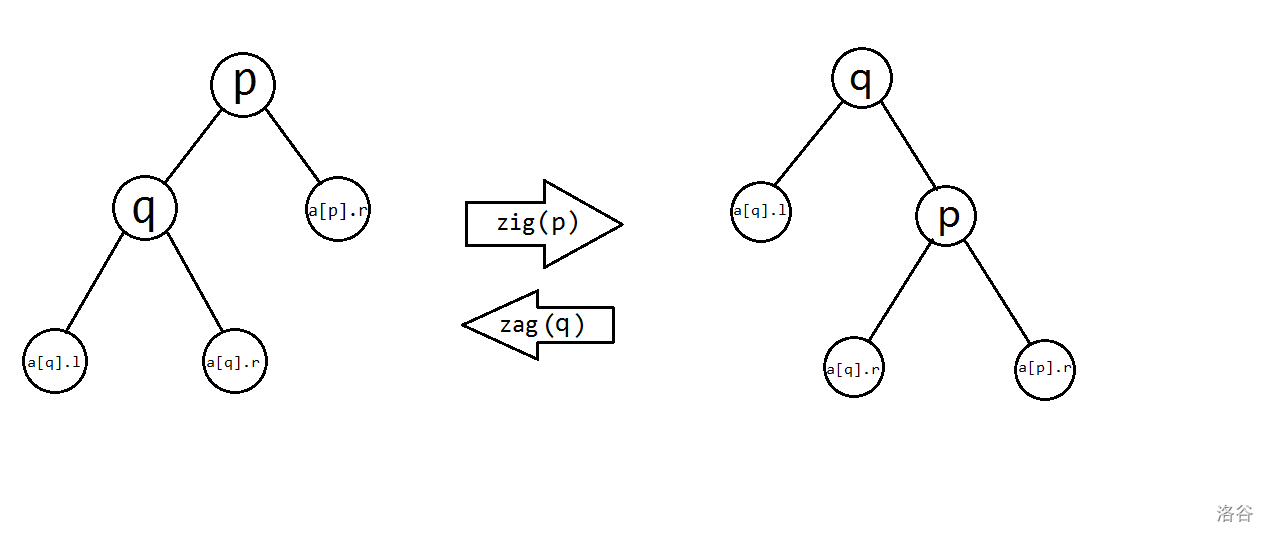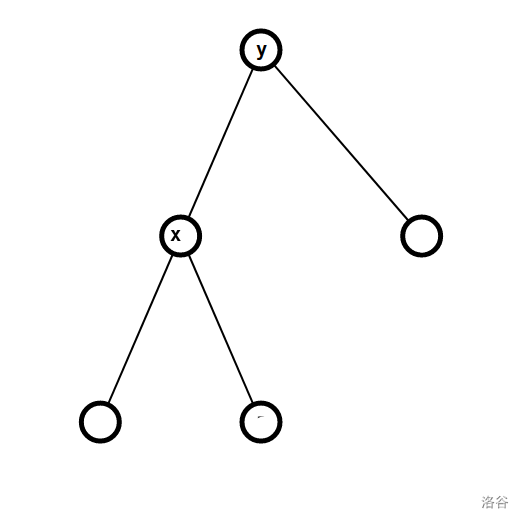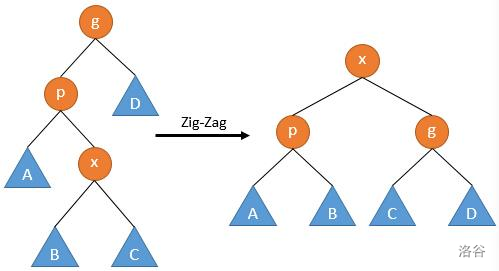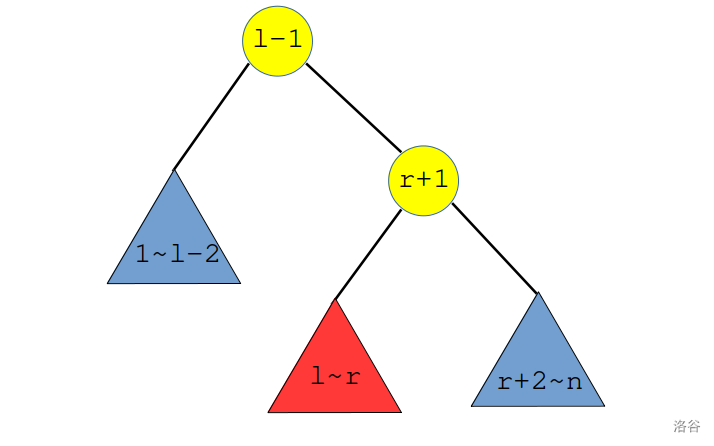# djwj233 的博客# djwj233 的博客

### Splay学习笔记

posted on 2021-08-05 19:17:57 | under 笔记 |

Splay 是一种平衡树，因此：

### Splay 的原理

Splay 是一种通过旋转维护平衡的平衡树。• 自己连到祖父（$\texttt{a[a[x].fa].fa}$）上；
• 将自己的右儿子连到原先的父亲上（也就是替代了自己）；
• 父亲连到自己上（也就是替代了右儿子）
void Splay::rotate(int x) {
int f=a[x].fa, c=which(x);
a[a[f].fa].ch[which(f)]=x, a[x].fa=a[f].fa;
a[f].ch[c]=a[x].ch[c^1], a[a[x].ch[c^1]].fa=f;
a[x].ch[c^1]=f, a[f].fa=x;
update(f), update(x); //注意一定要先更新f再更新x
}

（实际上，单旋 Splay 被称为 Spaly，是假算法，在HNOI2017中出现过）• 1/2 两种只需一次单旋 $\texttt{rotate(x)}$ 即可完成。
• 3/4 两种需要两次相同方向、不同节点的单旋 $\texttt{rotate(y)}$，$\texttt{rotate(x)}$ $\texttt{}$，被称为一字型旋转
• 5/6 两种需要两次不同方向、相同节if(a[f].fa) if(a[f].fa) if(a[f].fa) 点的单旋 $\texttt{rotate(x)}$，$\texttt{rotate(x)}$，被称为之字型旋转

void Splay::splay(int x) {
for( int f=a[x].fa ; f ; f=a[x].fa ) {
if(a[f].fa) rotate( which(x)==which(f) ? f : x );
rotate(x);
} rt=x;
}

（时间复杂度分析放在基本操作之后）

### Splay 的基本操作

• 单个结点

struct node {
int fa,ch;
int val;
int siz,cnt;
} a[N]; int tot,rt;
• 更新结点信息与基本操作

$\texttt{which}$ 操作询问了 $x$ 是其父亲的左结点还是右结点，这可以大大减少代码量。

int Splay::New(int v) {
a[++tot].val=v, a[tot].siz=a[tot].cnt=1;
}
void Splay::update(int x) {
a[x].siz=a[a[x].ch].siz+a[a[x].ch].siz+a[x].cnt;
}
int Splay::which(int x) {    return a[a[x].fa].ch==x;    }
• 单旋与双旋（重点）

• 插入

void Splay::insert(int &x,int f,int v)
{
if(x==0) {
x=New(v), a[x].fa=f;
splay(x); return ;
}
if(v<a[x].val) insert(a[x].ch,x,v);
else if(v==a[x].val) {
a[x].cnt++, splay(x);
return ;
}
else insert(a[x].ch,x,v);
}
• 查值与查排名
int Splay::getRank(int x,int v)
{
if(x==0) return 1;
if(v<a[x].val) return getRank(a[x].ch,v);
else if(v==a[x].val) {
int res=a[a[x].ch].siz+1;
splay(x); return res;
} else {
int res=a[a[x].ch].siz+a[x].cnt;
return res+getRank(a[x].ch,v);
}
}
int Splay::getVal(int x,int v)
{
if(x==0) {
splay(x); return a[x].val;
}
if(v<=a[a[x].ch].siz) return getVal(a[x].ch,v);
else if(v<=a[a[x].ch].siz+a[x].cnt) {
splay(x); return a[x].val;
} else return getVal(a[x].ch,v-a[a[x].ch].siz-a[x].cnt);
}
• 查前驱、后继

int Splay::getPre() {
int p=a[T.rt].ch;
while(a[p].ch) p=a[p].ch;
splay(p); return p;
}
int Splay::getNxt() {
int p=a[T.rt].ch;
while(a[p].ch) p=a[p].ch;
splay(p); return p;
}

T.insert(T.rt,0,x);
printf("%d\n",T.a[T.getPre()].val);
T.erase(x);
• 删除（重点）

1. 如果其 $cnt$ 值不小于 $2$，直接减去 $1$，然后 $\texttt{update}$；

2. 否则，如果它没有左儿子或没有右儿子，则直接用另一个儿子替换它；

3. 否则，将其左右儿子的两棵子树合并

那么怎么合并呢？我们把左子树中最大的数 $\texttt{splay}$ 上来当作新的根，那么原来的根一定在新根的右儿子处，直接将它删去即可。

void Splay::erase(int v){    getRank(T.rt,v);    if(a[T.rt].cnt>1) {        a[T.rt].cnt--, update(T.rt);        return ;    }    if(a[T.rt].ch==0) {        T.rt=a[T.rt].ch, a[T.rt].fa=0;        return ;    }    if(a[T.rt].ch==0) {        T.rt=a[T.rt].ch, a[T.rt].fa=0;         return ;    }    int cur=T.rt, x=getPre();    a[a[cur].ch].fa=T.        rt;    a[x].ch=a[cur].ch;    update(x);}

### Splay 的时间复杂度

##### 单旋##### 一字型旋转

• $\phi^\prime(x)=\phi(z)$；
• $\phi^\prime(z)\le\phi^\prime(y)\le\phi^\prime(x)$；
• $\phi(z)\ge\phi(y)\ge\phi(x)$。

• 引理：$\phi(x)+\phi^\prime(z)-2\phi^\prime(x)<-1$

##### 之字形旋转

$$\Delta \Phi\le \mathcal O(1)+\phi^\prime(x)+\phi^\prime(z)-2\phi(x)$$（图源这篇博客，其中 $p,g$ 分别等价于本文中的 $y,z$）

### Splay 的应用

##### 区间翻转void splay(int p,int t) { // t=0 or t=rt
for( int f=a[p].fa ; f!=t ; f=a[p].fa ) {
if(a[f].fa!=t) rotate( which(f)==which(p) ? f : p );
rotate(p);
}
if(a[p].fa==0) rt=p;
}

Code

Code